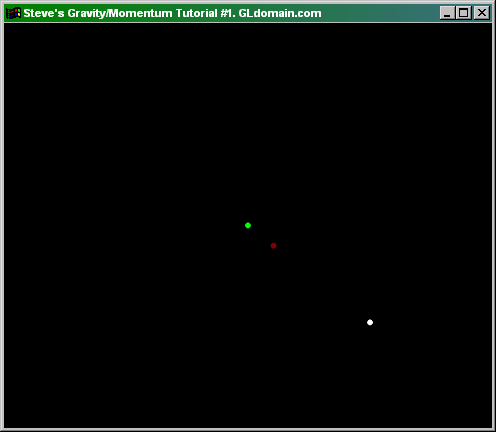You should also check to make sure Javascript is enabled.

Gravity/Momentum
Tutorial #1

When I say gravity, I don't mean the kind that makes all objects fall to the ground, but actually it refers to all objects attracted to each other.  You can use the particle engine for this effect, but we'll start a little simpler.

The easiest way to achieve attraction between objects is by using only 1 particle with an elastic attraction to the center of the screen:

 float x = 0; float y = 5; float xp = 5;  float yp = 0; float slowdown = 250; int DrawGLScene(GLvoid) // Here's Where We Do All The Drawing {   glClear(GL_COLOR_BUFFER_BIT | GL_DEPTH_BUFFER_BIT);   glLoadIdentity();   glTranslatef(0,0,-15);   xp += (0-x)/slowdown; //set xp according to distance from 0(elastic)   yp += (0-y)/slowdown; //set yp according to distance from 0(elastic)   x += xp/slowdown; // move x according to xp   y += yp/slowdown; // move y according to yp   glBegin(GL_POINTS);   glColor4f(1,1,1,1); // Set color to white   glVertex3f(x,y,0); // Draw pixel    glEnd(); }

As you may have noticed, elastic attraction is not what we want to achieve here.  There are ways to transform the algorithm into gravitational attraction.  The simplest way I have found that uses true-to-life physics is the following:

 double Reciprocal(double n) {    double close = .1f;   if (n < close && n >= 0) // prevent devision of 0   {     return 1/close;   }   else if (n > -close && n < 0) // prevent devision of 0   {     return 1/-close;   }    return 1/n;  } int DrawGLScene(GLvoid) // Here's Where We Do All The Drawing {   glClear(GL_COLOR_BUFFER_BIT | GL_DEPTH_BUFFER_BIT);   glLoadIdentity();   glTranslatef(0,0,-15);   Hypot = hypot(x,y);   if (x < 0)     angle = -acos(-y/-Hypot);   else     angle = acos(y/Hypot);   dist = Reciprocal(Hypot)/20;   xp -= sin(angle)*(dist); //set xp according to distance from 0(center of screen)   yp -= cos(angle)*(dist); //set yp according to distance from 0(center of screen)    x += xp/slowdown; // move x according to xp   y += yp/slowdown; // move y according to yp   glBegin(GL_POINTS);   glColor4f(1,1,1,1);    glVertex3f(x,y,0); // Draw pixel    glColor4f(1,0,0,dist*50);   glVertex3f(sin(angle),cos(angle),0); // Draw pixel    glColor4f(0,1,0,1);   glVertex3f(0,0,0); // Draw pixel    glEnd();   return TRUE; // Keep Going }

I'd like to explain a few things here.  First, the line dist = Reciprocal(Hypot)/20; is very important.  It is fundamental to allow a gravitational force instead of an elastic force.  Also, the angle variable is used to control the angle of force(much more advanced than the previous example).  Lastly, the xp and yp variables are now being controlled by the angle and the distThe algorithm is pretty complicated so instead of blabbing on and on like a math teacher, I'll give you an example program at this point:Hit Counter